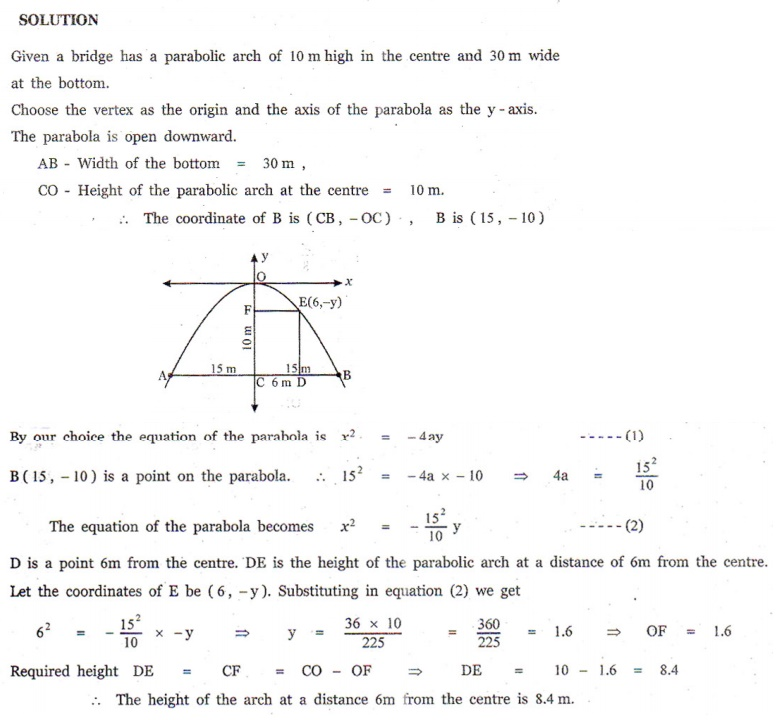Home | | Maths 12th Std | Exercise 5.5: Real life Applications of Conics

# Exercise 5.5: Real life Applications of Conics

Maths Book back answers and solution for Exercise questions - 1. Parabola 2. Ellipse 3. Hyperbola 4. Reflective property of parabola 5. Reflective Property of an Ellipse 6. Reflective Property of a Hyperbola - Exercise problems with Questions, Answers, Solution, Explanation

EXERCISE 5.5

1. A bridge has a parabolic arch that is 10high in the centre and 30wide at the bottom. Find the height of the arch 6from the centre, on either sides.2. A tunnel through a mountain for a four lane highway is to have a elliptical opening. The total width of the highway (not the opening) is to be 16m, and the height at the edge of the road must be sufficient for a truck 4high to clear if the highest point of the opening is to be 5approximately . How wide must the opening be?3. At a water fountain, water attains a maximum height of 4at horizontal distance of 0.5from its origin. If the path of water is a parabola, find the height of water at a horizontal distance of 0.75from the point of origin.4. An engineer designs a satellite dish with a parabolic cross section. The dish is 5wide at the opening, and the focus is placed 1.2from the vertex

(a)  Position a coordinate system with the origin at the vertex and the x -axis on the parabola’s axis of symmetry and find an equation of the parabola.

(b)  Find the depth of the satellite dish at the vertex.5. Parabolic cable of a 60portion of the roadbed of a suspension bridge are positioned as shown below. Vertical Cables are to be spaced every 6malong this portion of the roadbed. Calculate the lengths of first two of these vertical cables from the vertex.6. Cross section of a Nuclear cooling tower is in the shape of a hyperbola with equation.

The tower is 150m tall and the distance from the top of the tower to the centre of the hyperbola is half the distance from the base of the tower to the centre of the hyperbola. Find the diameter of the top and base of the tower.7. A rod of length 1.2moves with its ends always touching the coordinate axes. The locus of a point on the rod, which is 0.3from the end in contact with -axis is an ellipse. Find the eccentricity.8. Assume that water issuing from the end of a horizontal pipe, 7.5above the ground, describes a parabolic path. The vertex of the parabolic path is at the end of the pipe. At a position 2.5 below the line of the pipe, the flow of water has curved outward 3beyond the vertical line through the end of the pipe. How far beyond this vertical line will the water strike the ground?9. On lighting a rocket cracker it gets projected in a parabolic path and reaches a maximum height of 4when it is 6away from the point of projection. Finally it reaches the ground 12away from the starting point. Find the angle of projection.10. Points and are 10km apart and it is determined from the sound of an explosion heard at those points at different times that the location of the explosion is 6 km closer to than . Show that the location of the explosion is restricted to a particular curve and find an equation of it.